Publicité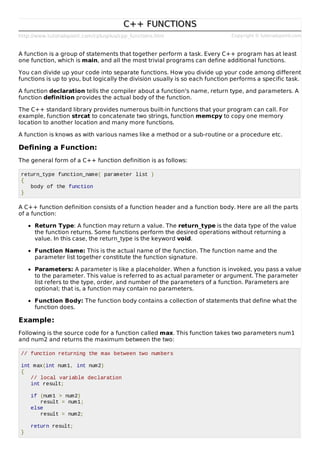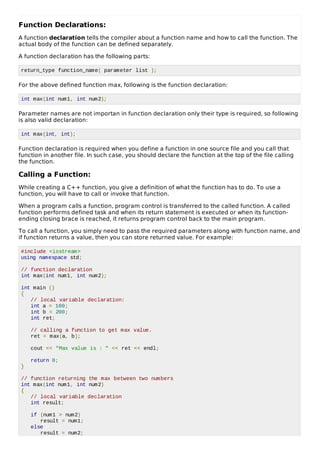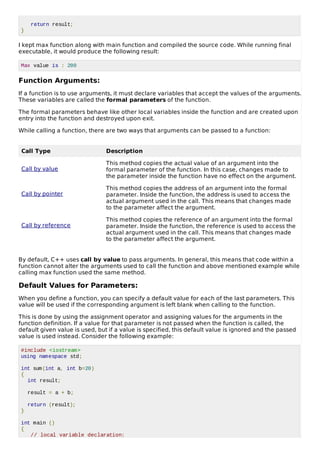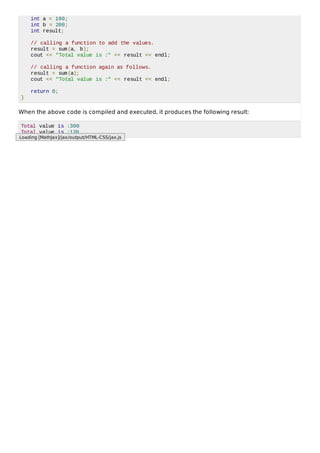Prochain SlideShare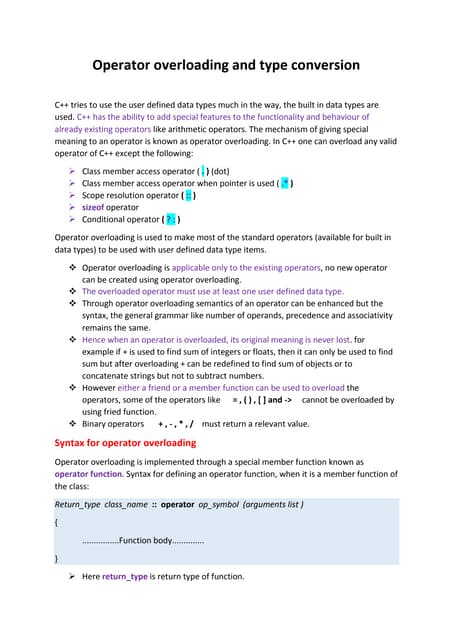M11 operator overloading and type conversion
Chargement dans ... 3
1 sur 4
Publicité

### Cpp functions

1. http://www.tutorialspoint.com/cplusplus/cpp_functions.htm Copyright © tutorialspoint.com C++ FUNCTIONS C++ FUNCTIONS A function is a group of statements that together perform a task. Every C++ program has at least one function, which is main, and all the most trivial programs can define additional functions. You can divide up your code into separate functions. How you divide up your code among different functions is up to you, but logically the division usually is so each function performs a specific task. A function declaration tells the compiler about a function's name, return type, and parameters. A function definition provides the actual body of the function. The C++ standard library provides numerous built-in functions that your program can call. For example, function strcat to concatenate two strings, function memcpy to copy one memory location to another location and many more functions. A function is knows as with various names like a method or a sub-routine or a procedure etc. Defining a Function: The general form of a C++ function definition is as follows: return_type function_name( parameter list ) { body of the function } A C++ function definition consists of a function header and a function body. Here are all the parts of a function: Return Type: A function may return a value. The return_type is the data type of the value the function returns. Some functions perform the desired operations without returning a value. In this case, the return_type is the keyword void. Function Name: This is the actual name of the function. The function name and the parameter list together constitute the function signature. Parameters: A parameter is like a placeholder. When a function is invoked, you pass a value to the parameter. This value is referred to as actual parameter or argument. The parameter list refers to the type, order, and number of the parameters of a function. Parameters are optional; that is, a function may contain no parameters. Function Body: The function body contains a collection of statements that define what the function does. Example: Following is the source code for a function called max. This function takes two parameters num1 and num2 and returns the maximum between the two: // function returning the max between two numbers int max(int num1, int num2) { // local variable declaration int result; if (num1 > num2) result = num1; else result = num2; return result; }
2. Function Declarations: A function declaration tells the compiler about a function name and how to call the function. The actual body of the function can be defined separately. A function declaration has the following parts: return_type function_name( parameter list ); For the above defined function max, following is the function declaration: int max(int num1, int num2); Parameter names are not importan in function declaration only their type is required, so following is also valid declaration: int max(int, int); Function declaration is required when you define a function in one source file and you call that function in another file. In such case, you should declare the function at the top of the file calling the function. Calling a Function: While creating a C++ function, you give a definition of what the function has to do. To use a function, you will have to call or invoke that function. When a program calls a function, program control is transferred to the called function. A called function performs defined task and when its return statement is executed or when its function- ending closing brace is reached, it returns program control back to the main program. To call a function, you simply need to pass the required parameters along with function name, and if function returns a value, then you can store returned value. For example: #include <iostream> using namespace std; // function declaration int max(int num1, int num2); int main () { // local variable declaration: int a = 100; int b = 200; int ret; // calling a function to get max value. ret = max(a, b); cout << "Max value is : " << ret << endl; return 0; } // function returning the max between two numbers int max(int num1, int num2) { // local variable declaration int result; if (num1 > num2) result = num1; else result = num2;
3. return result; } I kept max function along with main function and compiled the source code. While running final executable, it would produce the following result: Max value is : 200 Function Arguments: If a function is to use arguments, it must declare variables that accept the values of the arguments. These variables are called the formal parameters of the function. The formal parameters behave like other local variables inside the function and are created upon entry into the function and destroyed upon exit. While calling a function, there are two ways that arguments can be passed to a function: Call Type Description Call by value This method copies the actual value of an argument into the formal parameter of the function. In this case, changes made to the parameter inside the function have no effect on the argument. Call by pointer This method copies the address of an argument into the formal parameter. Inside the function, the address is used to access the actual argument used in the call. This means that changes made to the parameter affect the argument. Call by reference This method copies the reference of an argument into the formal parameter. Inside the function, the reference is used to access the actual argument used in the call. This means that changes made to the parameter affect the argument. By default, C++ uses call by value to pass arguments. In general, this means that code within a function cannot alter the arguments used to call the function and above mentioned example while calling max function used the same method. Default Values for Parameters: When you define a function, you can specify a default value for each of the last parameters. This value will be used if the corresponding argument is left blank when calling to the function. This is done by using the assignment operator and assigning values for the arguments in the function definition. If a value for that parameter is not passed when the function is called, the default given value is used, but if a value is specified, this default value is ignored and the passed value is used instead. Consider the following example: #include <iostream> using namespace std; int sum(int a, int b=20) { int result; result = a + b; return (result); } int main () { // local variable declaration:
4. int a = 100; int b = 200; int result; // calling a function to add the values. result = sum(a, b); cout << "Total value is :" << result << endl; // calling a function again as follows. result = sum(a); cout << "Total value is :" << result << endl; return 0; } When the above code is compiled and executed, it produces the following result: Total value is :300 Total value is :120 Loading [MathJax]/jax/output/HTML-CSS/jax.js
Publicité